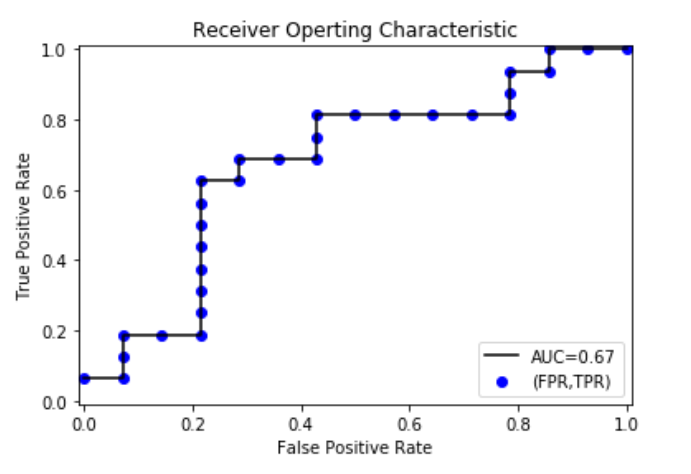# AUC(Area Under Curve)

## ROC与AUC常用来评价一个二值分类器(binary classifier)的优劣

### 1.ROC曲线：

#### 只讨论二值分类器。对于分类器，或者说分类算法，评价指标主要有precision，recall，F-score，以及ROC和AUC。下图是一个ROC曲线的示例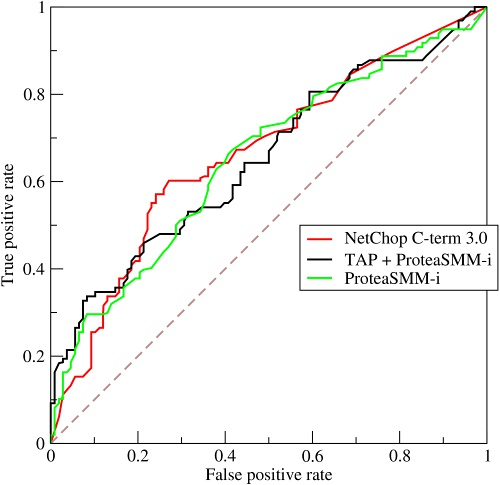#### TPR：实例标签为1，被预测为正确的比例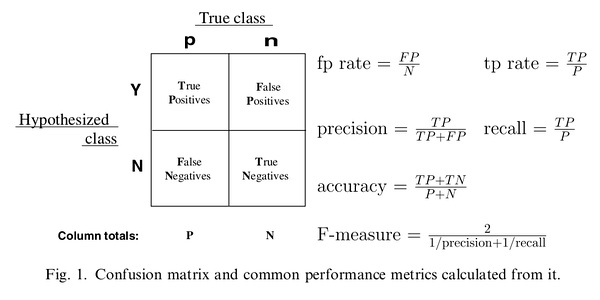#### 混淆矩阵：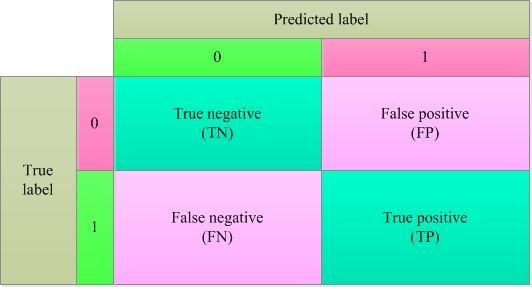### 2.绘制ROC曲线

#### 我们需要选定阈值才能把概率转化为类别，如果我们选定阈值为0.1，那么5个样本被分进1的类别，如果选定0.3，结果仍然一样，如果选了0.45作为阈值那么只有样本4被分进0，其余的都进入1类，就可以计算FTR,PTR，把所有得到的所有FTR,PTR绘成线，就可以得到ROC曲线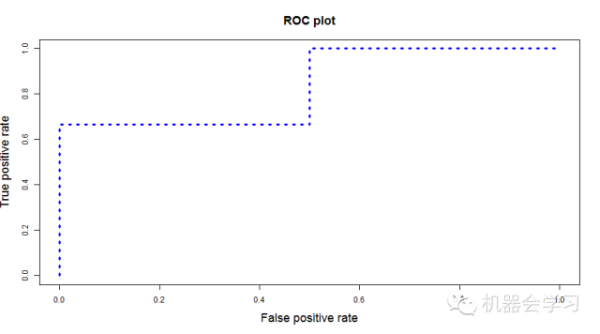#### 我们根据每个测试样本属于正样本的概率值从大到小排序。下图是一个示例，图中共有20个测试样本，“Class”一栏表示每个测试样本真正的标签（p表示正样本，n表示负样本），“Score”表示每个测试样本属于正样本的概率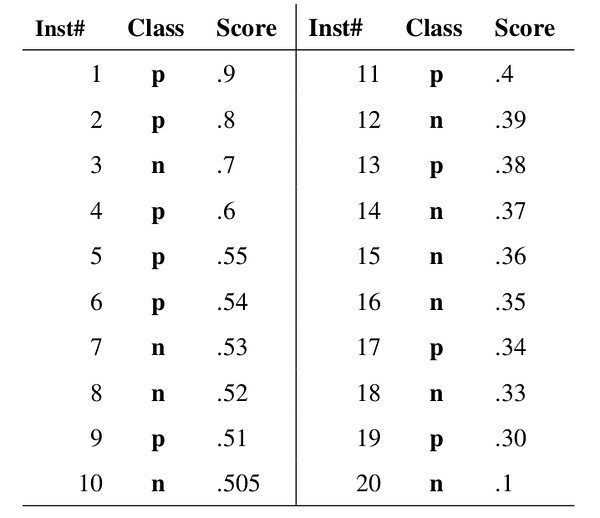#### 我们从高到低，依次将“Score”值作为阈值threshold，当测试样本属于正样本的概率大于或等于这个threshold时，我们认为它为正样本，否则为负样本。举例来说，对于图中的第4个样本，其“Score”值为0.6，那么样本1，2，3，4都被认为是正样本，因为它们的“Score”值都大于等于0.6，而其他样本则都认为是负样本。每次选取一个不同的threshold，我们就可以得到一组FPR和TPR，即ROC曲线上的一点。这样一来，我们一共得到了20组FPR和TPR的值，将它们画在ROC曲线的结果如下图：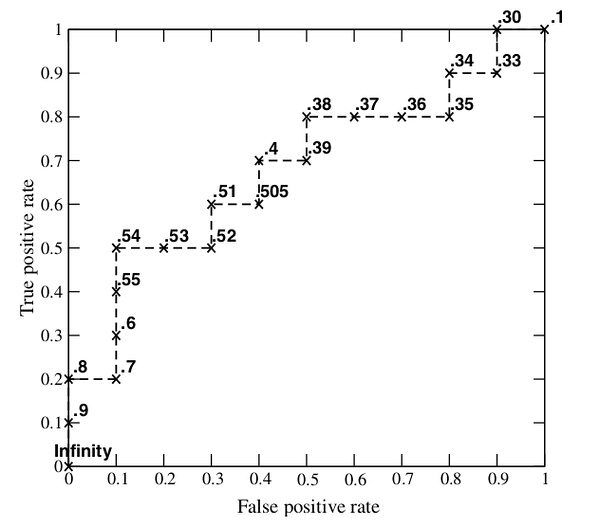### 3.AUC

#### ROC曲线有个很好的特性：当测试集中的正负样本的分布变化的时候，ROC曲线能够保持不变。在实际的数据集中经常会出现类不平衡（class imbalance）现象，即负样本比正样本多很多（或者相反），而且测试数据中的正负样本的分布也可能随着时间变化。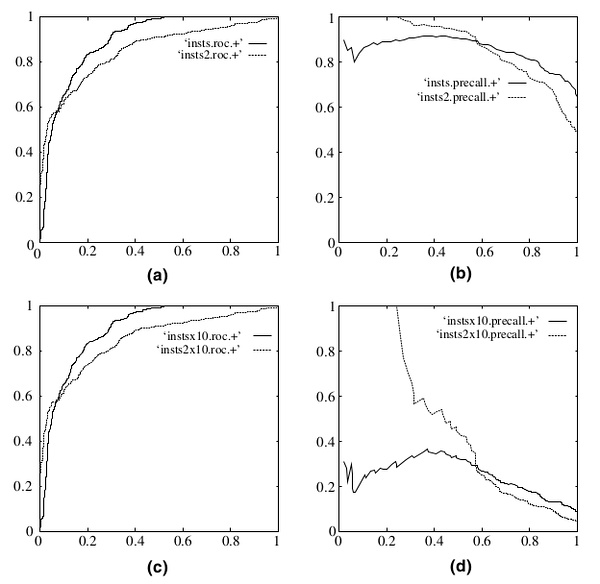### 4.计算代码

import numpy as np
import pandas as pd
import matplotlib.pyplot as plt
%matplotlib inline

parameter = 30
data = pd.DataFrame(index = range(0,parameter),columns = ('probability','The true label'))
data['The true label'] = np.random.randint(0,2,size = len(data))
data['probability'] = np.random.choice(np.arange(0.1,1,0.1),len(data['probability']))
data#random库随机生成数据

cm = np.arange(4).reshape(2,2)
cm[0,0] = len(data[data['The true label']==0][data['probability']<0.5])#TN
cm[0,1] = len(data[data['The true label']==0][data['probability']>=0.5])#FP
cm[1,0] = len(data[data['The true label']==1][data['probability']<0.5])#FN
cm[1,1] = len(data[data['The true label']==1][data['probability']>=0.5])#TP

import itertools#自带
classes = [0,1]
plt.figure()
plt.imshow(cm,interpolation='nearest',cmap=plt.cm.Blues)
plt.title('Confusion matrix')
#画方块
tick_marks = np.arange(len(classes))
plt.xticks(tick_marks,classes,rotation = 0)
plt.yticks(tick_marks,classes)
thresh = cm.max()/2
for i,j in itertools.product(range(cm.shape),range(cm.shape)):
plt.text(j,i,cm[i,j],horizontalalignment='center',color='white' if cm[i,j]>thresh else 'black')
plt.tight_layout()
plt.ylabel('True label')
plt.xlabel('Predicted label')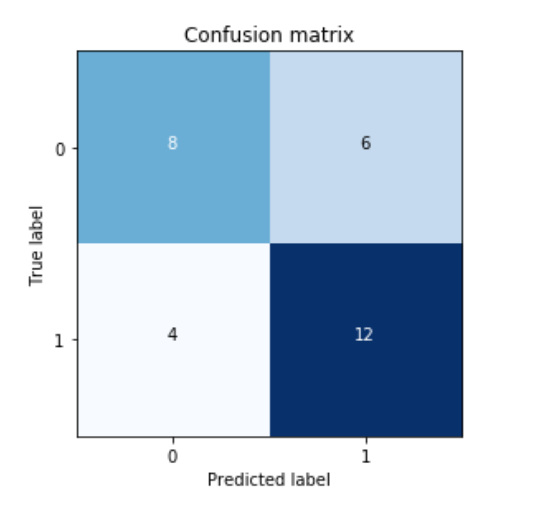data.sort_values('probability',inplace=True, ascending=False)#排序
data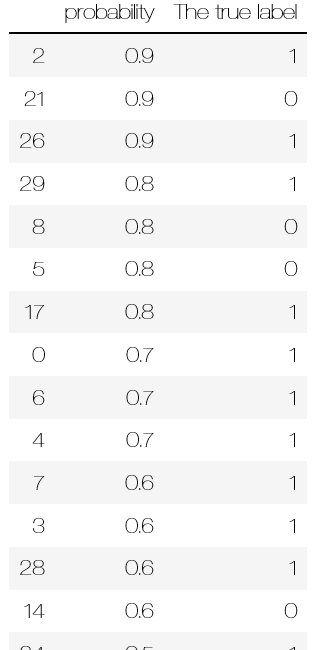TPRandFPR = pd.DataFrame(index=range(len(data)),columns=('TP','FP'))
for j in range(len(data)):
FP=len(data1[data1['The true label']==0][data1['probability']>=data1.head(len(data1))['probability']])/float(len(data[data['The true label']==0]))
TP=len(data1[data1['The true label']==1][data1['probability']>=data1.head(len(data1))['probability']])/float(len(data[data['The true label']==1]))
TPRandFPR.iloc[j]=[TP,FP]
TPRandFPR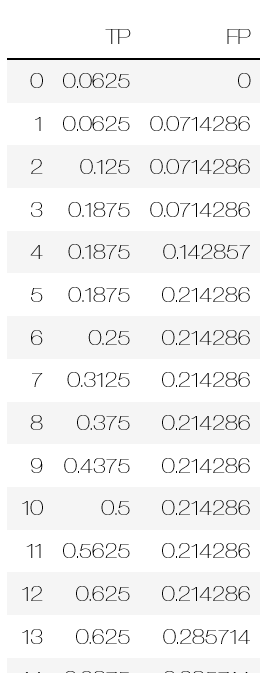from sklearn.metrics import auc
#threshold依次取0.9,0.9,0.9,0.8……
AUC = auc(TPRandFPR['FP'],TPRandFPR['TP'])
plt.scatter(x=TPRandFPR['FP'],y=TPRandFPR['TP'],label='(FPR,TPR)',color='b')
plt.plot(TPRandFPR['FP'],TPRandFPR['TP'],'k',label='AUC=%0.2f'%AUC)
plt.legend(loc='lower right')
plt.show()